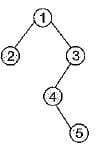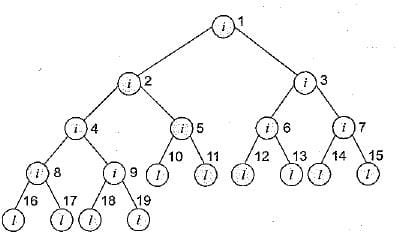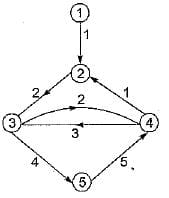# Test: Graphs & Hashing- 1

## 10 Questions MCQ Test Question Bank for GATE Computer Science Engineering | Test: Graphs & Hashing- 1

Description
Attempt Test: Graphs & Hashing- 1 | 10 questions in 30 minutes | Mock test for Computer Science Engineering (CSE) preparation | Free important questions MCQ to study Question Bank for GATE Computer Science Engineering for Computer Science Engineering (CSE) Exam | Download free PDF with solutions
QUESTION: 1

### The average search time of hashing, with linear probing will be less if the load factor

Solution:

Load factor is the ratio of number of records that are currently present and total number of records that can be present. If the load factor is less, free space will be more. This means probability of collision is less. So, the search time will be less.

QUESTION: 2

### A hash table can store a maximum of 10 records. Currently there are records in locations 1, 3, 4, 7, 8, 9, 10. The probability of a new record going into location 2, with a has function resolving collisions by linear probing is

Solution:

If the new record hashes onto one of the six locations 7, 8, 9, 10, 1 or 2, the location 2 will receive the new record. The probability is 6/10 (as 10 is the total possible number of locations).

QUESTION: 3

### Consider a hashing function that resolves collision by quadratic probing. Assume the address space is indexed from 1 to 8. Which of the following locations will never be probed if a collision occurs at position 4?

Solution:

You can verify that the 1st, 3rd, 5th, 7th...probes check at location 5.
The 2nd, 6th, 10th...probes check at location 8. The 4th, 8th, 12th...probes check at location 4. The rest of the address space, will never be probed.

QUESTION: 4

A has table has space for 100 records. What is the probability of collision before the table is 10% full?

Solution:

If there is only one record, then the probability of a collision will be 1/100. if 2, then 2/100 etc. If 9 then 9/100. So, the required probability is 1 + 2 + 3 ... 9/100 = 0.45.

QUESTION: 5

A hash function randomly distributes records one by one in a space that can hold x number of records. The probability that the mth record is the first record to result in collision is

Solution:

Probability for the first record not colliding is x/x.
Probability for the second record not colliding is x - 1/x. (This is because one place is already occupied. So favorable number of cases is x-1).
Probability for the third record not colliding is x - 2/x.
Probability for the (m - 1)th record not colliding is x - (m - 2)/x.
Now the next (mth) record is resulting in a collision. Out of the x places, it should hash to one of the (m - 1) places already, filled. So probability is (m - 1)/x.
The required probability is (x/x) (x - 1/x) (x - 2/x) ...(x - (m - 2)/x) (m - 1/x)

QUESTION: 6

In the given binary tree, using array (the array starts from index 1), you can store the node 4 at location,Solution:

Since, 4 is the left child of 3 and 3 have index value 3. So, 4 has index value 2 x [index value of parent 3]
= 2 x 3 = 6

QUESTION: 7

A binary tree in which every non-leaf node has non-empty left and right subtrees is called a strictly binary tree. Such a tree with 10 leaf nodes

Solution:

10 leaf nodes, where i = internal node and l = leaf nodeSo number of nodes in strict binary tree will be:
Nodes = n + (n - 1), n = leaf, (n - 1) internal
= (2n - 1)
So = 10 + (10 - 1)
= 10 + 9
= 19

QUESTION: 8

The eccentricity of node labeled 5 in the graph inSolution:

Eccentricity of a given node is the maximum of minimum path from other nodes to the given node.
Cost of minimum path from 1 to 5 is 7 Cost of minimum path from 2 to 5 is 6 Cost of minimum path from 3 to 5 is 4 Cost of minimum path from 4 to 5 is 7 Since the maximum is 7, eccentricity of node 5 is 7.

QUESTION: 9

The center of the graph in above questions is the node labeled

Solution:

Find eccentricity of all nodes.
Eccentricity of node 1 is ∞
Eccentricity of node 2 is 6
Eccentricity of node 3 is 8
Eccentricity of node 4 is 5
Eccentricity of node 5 is 7
Center of a graph is the node with minimum eccentricity.

QUESTION: 10

In which of the following cases, linked list implementations of sparse matrices consumes the same memory space as the conventional way of storing the entire array?
(Assume all data-types need the same amount of storage.)

Solution:

Conventional way needs a storage of m x n.
In the case of linked list implementation of sparse matrices, storage needed will be m + 3 x (the number of non-zero entries).
Only in case (c), both the methods need the same storage of 30.Use Code STAYHOME200 and get INR 200 additional OFF Use Coupon Code